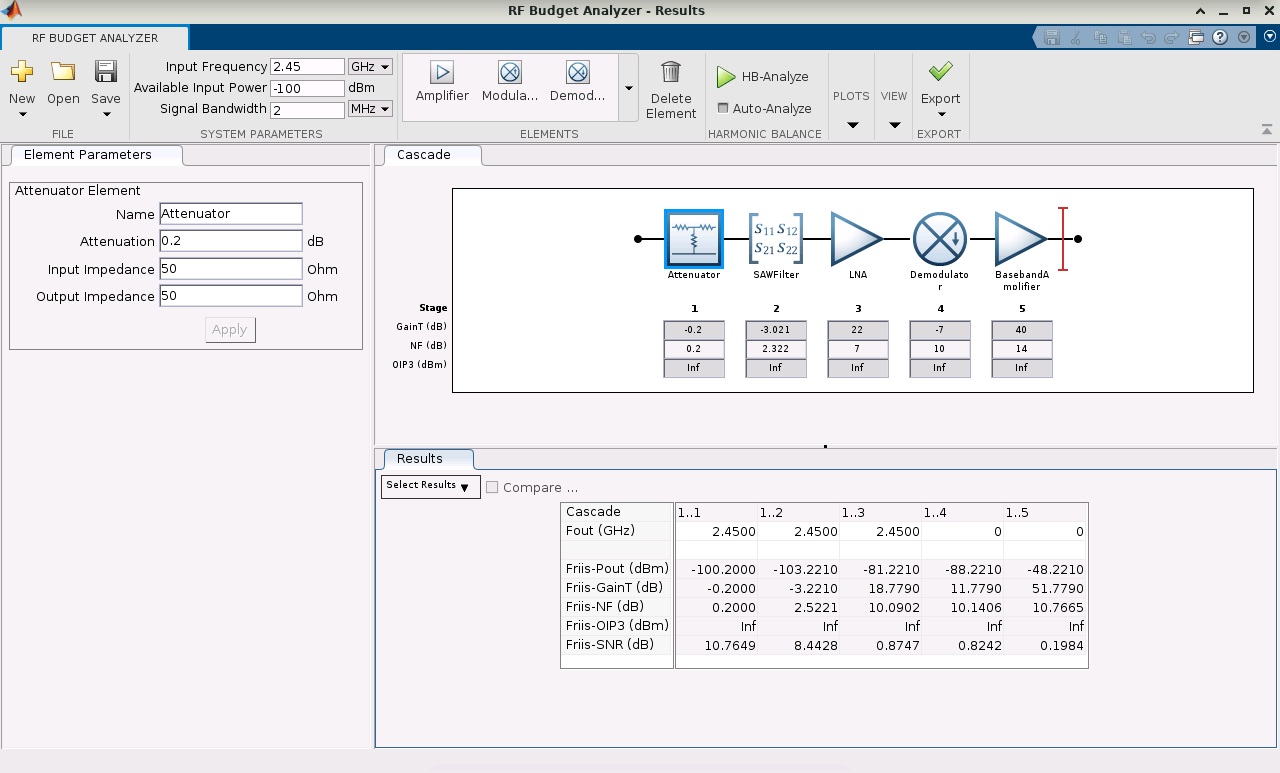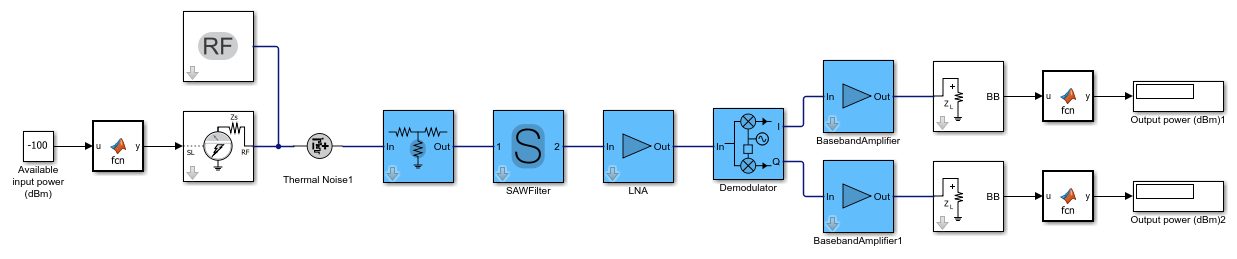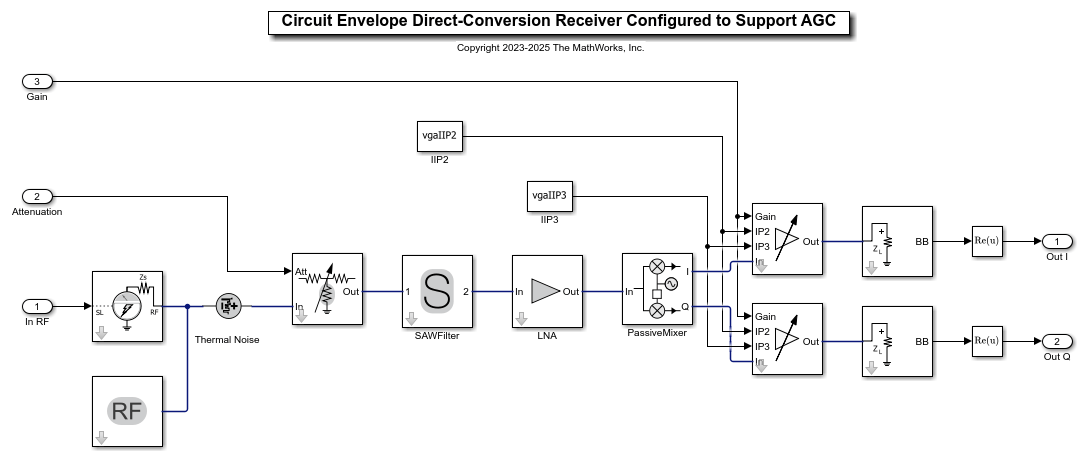This example shows how to design an RF direct-conversion receiver (DCR) that supports variable gain and attenuation control. The design workflow entails the following steps:

2. Export the RF budget to RF Blockset™.

3. Make further modifications to the circuit envelope model.

4. Simulate and test the model behavior.

### Design Receiver in RF Budget Analyzer App

You can model the DCR with an RF chain that has the following stages:

• Attenuator — Prevents saturation and overload of the RF front-end by strong incoming signals that can desensitize or damage the receiver

• Surface acoustic wave (SAW) filter — Performs RF band selection

• Low-noise amplifier (LNA) — Amplifies the received RF signal without significantly degrading the signal-to-noise ratio (SNR)

• Demodulator — Downconverts the RF signal to baseband

• Baseband amplifier — Regulates the output signal power, for example, to keep it within the dynamic range of a downstream analog-to-digital converter (ADC)

Typically, a low-pass filter is appended to the end of the RF chain for the DCR to extract the baseband signal within the band of interest, that is, to perform channel selection. For the RF budget analysis here, assume ideal channel-selection filtering at the output of the demodulator.

Design this RF chain either interactively with the RF Budget Analyzer app or progammatically with `circuit` and `rfbudget` objects.

```RFDCRBudget = rfbudget([ ... attenuator(Name='Attenuator',Attenuation=0.2), ... nport(Name='SAWFilter',FileName='SAW_Filter_Data.s2p'), ... amplifier(Name='LNA',Gain=22,NF=7), ... modulator(Name='Demodulator',ConverterType='Down',LO=2.45e9,Gain=-7,NF=10), ... amplifier(Name='BasebandAmplifier',Gain=40,NF=14)], ... InputFrequency=2.45e9, ... AvailableInputPower=-100, ... SignalBandwidth=2e6) ```
```RFDCRBudget = rfbudget with properties: Elements: [1x5 rf.internal.rfbudget.Element] InputFrequency: 2.45 GHz AvailableInputPower: -100 dBm SignalBandwidth: 2 MHz Solver: Friis AutoUpdate: true Analysis Results OutputFrequency: (GHz) [ 2.45 2.45 2.45 0 0] OutputPower: (dBm) [-100.2 -103.2 -81.22 -88.22 -48.22] TransducerGain: (dB) [ -0.2 -3.221 18.78 11.78 51.78] NF: (dB) [ 0.2 2.522 10.09 10.14 10.77] IIP2: (dBm) [] OIP2: (dBm) [] IIP3: (dBm) [ Inf Inf Inf Inf Inf] OIP3: (dBm) [ Inf Inf Inf Inf Inf] SNR: (dB) [ 10.76 8.443 0.8747 0.8242 0.1984] ```

Execute the following command to visualize the design of the RF chain and its budget analysis in the RF Budget Analyzer app.

```rfBudgetAnalyzer(RFDCRBudget) ```### Export RF Budget to RF Blockset to Automatically Generate Circuit Envelope Model

The circuit envelope model supports multicarrier simulation of the DCR while taking into account nonlinear effects and impairments, mixer phase noise and LO-RF isolation, and the impact of and interactions between the signal of interest and other in-band and out-of-band interfering signals.

Create a circuit envelope model from the `rfbudget` object or interactively from within the RF Budget Analyzer app.

```exportRFBlockset(RFDCRBudget) ```As seen in the automatically generated model, this example makes the following assumptions:

• In the Configuration block, the simulation step size is automatically set to 1/8 times the reciprocal of the input signal envelope bandwidth. In this example, this input signal bandwidth is 2 MHz and is centered around the input carrier frequency of 2.45 GHz. This corresponds to a simulation step size of 62.5 ns and a simulation bandwidth of 16 MHz.

• Like that of the budget analysis, the demodulator is configured to perform ideal low-pass channel-selection filtering within the simulation bandwidth around the output carrier of interest, which is at DC (0 Hz). Although it is out of scope for this example, you can modify the channel-selection filter options in the IQ Demodulator block to model realistic and realizable channel-selection designs and implementations.

• The demodulator is implemented with a quadrature architecture, which is a standard modeling paradigm when the signal of interest is QPSK-modulated and contains both in-phase (I) and quadrature (Q) components. It is standard modeling practice to combine the two extracted, real-valued baseband signals, representing the two components as a single complex-valued baseband signal (I+jQ) and feeding this signal into the baseband QPSK demodulator.

The S-Parameters block describing the SAW filter uses rational fitting in order to simulate frequency data in the time domain. Note that at 2.45 GHz the filter introduces a phase rotation of approximately –59 degrees. While it is not shown here, if you simulate this model, you can see that the complex input signal is partly downconverted on the I and Q branches, and thus the instantaneous output powers on the two branches can be different. However, the time-averaged output powers on the I and Q branches are expected to be the same. In addition, the average of the I and Q instantaneous output powers, that is (I(t)+Q(t))/2, is expected to match the output power reported in the budget analysis.

### Configure Model to Support Automatic Gain Control

Make the following changes to the model to prepare it for use within an automatic gain control (AGC) loop:

• Replace the Attenuator block with a Variable Attenuator block. Control the variable attenuation using one of the model's root-level input ports.

• Replace the Amplifier blocks with VGA blocks. Control the variable gain using one of the model's root-level input ports.

• Assume that the IP2 and IP3 values for each VGA block are gain independent. Accordingly, specify them as constant values, and configure the VGA block to use the input-referred convention for the intercept points: IIP2 and IIP3. If you want to specify gain-dependent IP2 and IP3 values, then you can configure the VGA block to use the output-referred convention for the intercept points and then use lookup table blocks to specify the OIP2 vs. Gain and OIP3 vs. Gain relations. Note that this is out of scope for this example.

• Configure the RF Outport block to output complex baseband signals for I and Q each, and take the real part of each signal for the purposes of data type conversion. Note that the imaginary part of each signal is zero. Typically, a real passband representation is the more appropriate choice, given that the output signal is at DC (0 Hz). However, this model uses frame-based input signals to accelerate simulation time, and the RF Blockset Circuit Envelope library does not support frame-based processing when the Outport block's Output is set to `Real Passband`. Although this example does not explicitly show it, you can confirm that each I and Q signal has zero imaginary part.

• Set Samples per frame to `256` in the Configuration block to help accelerate simulation time.

• Configure the signal attributes of the model's root-level input, specifically the one corresponding to the input RF signal `In RF`, to be of complex-valued signal type. This input RF signal is a complex equivalent baseband signal, and it corresponds to the envelope of the RF carrier, which is at 2.45 GHz.

Specify amplifier nonlinearities for the VGA blocks.

```vgaIIP2 = 50; vgaIIP3 = 30; ```

Specify mixer impairments for the IQ Demodulator block.

```mixerParameters.LOToRFIsolation = 105; mixerParameters.PhaseNoiseFrequencyOffsets = 1e6*[0.2 1 2 3 7]; mixerParameters.PhaseNoiseLevels = [-70 -80 -95 -110 -120]; mixerParameters.IIP2 = 55; mixerParameters.CSFilterPassBandEdgeFrequency = 50e6; ```

View the final model.

```open_system RFDCR ```